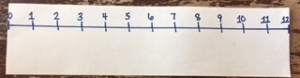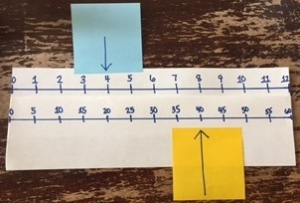Telling a time is an important concept for everyday life. It’s also one that students some time struggle with if they don’t understand how a clock works.

In 3rd grade, students are expected to:
• Tell and write time to the nearest minute
• Use analog and digital clocks to tell time
• Measure time intervals in minutes
• Solve word problems that involve addition and subtraction of time intervals in minutes and represent the problems on a number line.

Using a linear number line model can sometimes help with misconceptions students have about telling time. Here are some ideas on how you can relate a number line and a clock to help students tell time:

Explore Time in a Number line format to encourage skip counting.
1. Create a number line with the intervals for the hours on a clock.2. Create a number line with the intervals for the minutes on a clock.3. Compare the two number lines and encourage students to look for patterns.4. Pose questions for students to compare how hours and minutes relate and work on a clock, such as:
o How many minutes relate to the 3rd hour?
o How many hours relate to 40 minutes?
o What pattern do you notice on the minute hand number line?
o What pattern do you notice on the hour hand number line?

5. Extend to show an hour hand on the hour number line and a minute hand on the minute number line. Have the students determine the time and record it digitally.6. This could also be done by making the linear clock into a circular clock. Use index cards to record each hour. Then attach the times with string to change it to a circular clock. Then explore time by just using the hour hand to determine time to the nearest and time to the nearest half hour. Could also include the minute and hour hand to use patterns to determine the time.For more information on relating a Number Line to a Clock, visit https://learnzillion.com/resources/64146-relating-skip-counting-to-time.